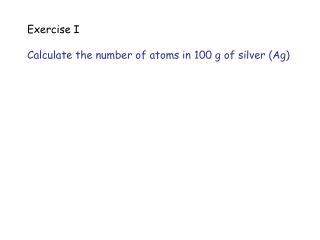DownloadDownload PresentationExercise I Calculate the number of atoms in 100 g of silver (Ag)

# Exercise I Calculate the number of atoms in 100 g of silver (Ag)

Télécharger la présentation## Exercise I Calculate the number of atoms in 100 g of silver (Ag)

- - - - - - - - - - - - - - - - - - - - - - - - - - - E N D - - - - - - - - - - - - - - - - - - - - - - - - - - -
##### Presentation Transcript

1. Exercise I Calculate the number of atoms in 100 g of silver (Ag)

2. Exercise II Plot the melting temperatures of elements in the 4A to 8–10 columns of the periodic table versus atomic number (i.e., plot melting temperatures of Ti through Ni, Zr through Pd, and Hf through Pt). Discuss these relationships, based on atomic bonding and binding energy, (a) as the atomic number increases in each row of the periodic table and (b) as the atomic number increases in each column of the periodic table.

3. Solution: For each row, the melting temperature is highest when the outer “d” energy level is partly full. In Cr, there are 5 electrons in the 3d shell; in Mo, there are 5 electrons in the 4d shell; in W there are 4 electrons in the 5d shell. In each column, the melting temperature increases as the atomic number increases—the atom cores contain a larger number of tightly held electrons, making the metals more stable.

4. Exercise III Plot the melting temperature of the elements in the 1A column of the periodic table versus atomic number (i.e., plot melting temperatures of Li through Cs). Discuss this relationship, based on atomic bonding and binding energy.

5. Solution: As the atomic number increases, the melting temperature decreases, opposite with previous exercise.

6. HAVE A NICE WEEKEND QUIZ ON MONDAY website for notes:http://www.mae.wvu.edu/~cairns/courses.html username: cairns password: materials